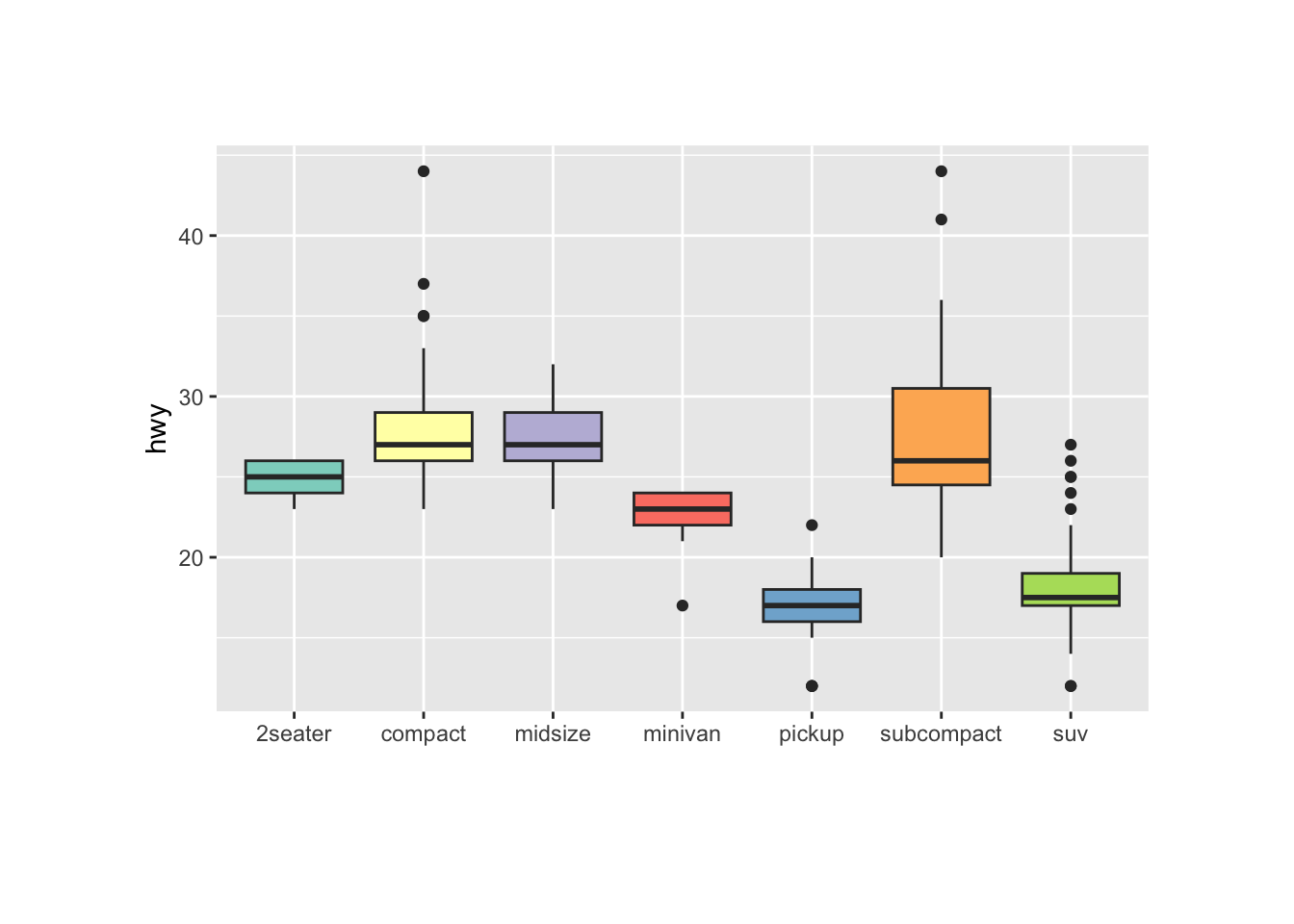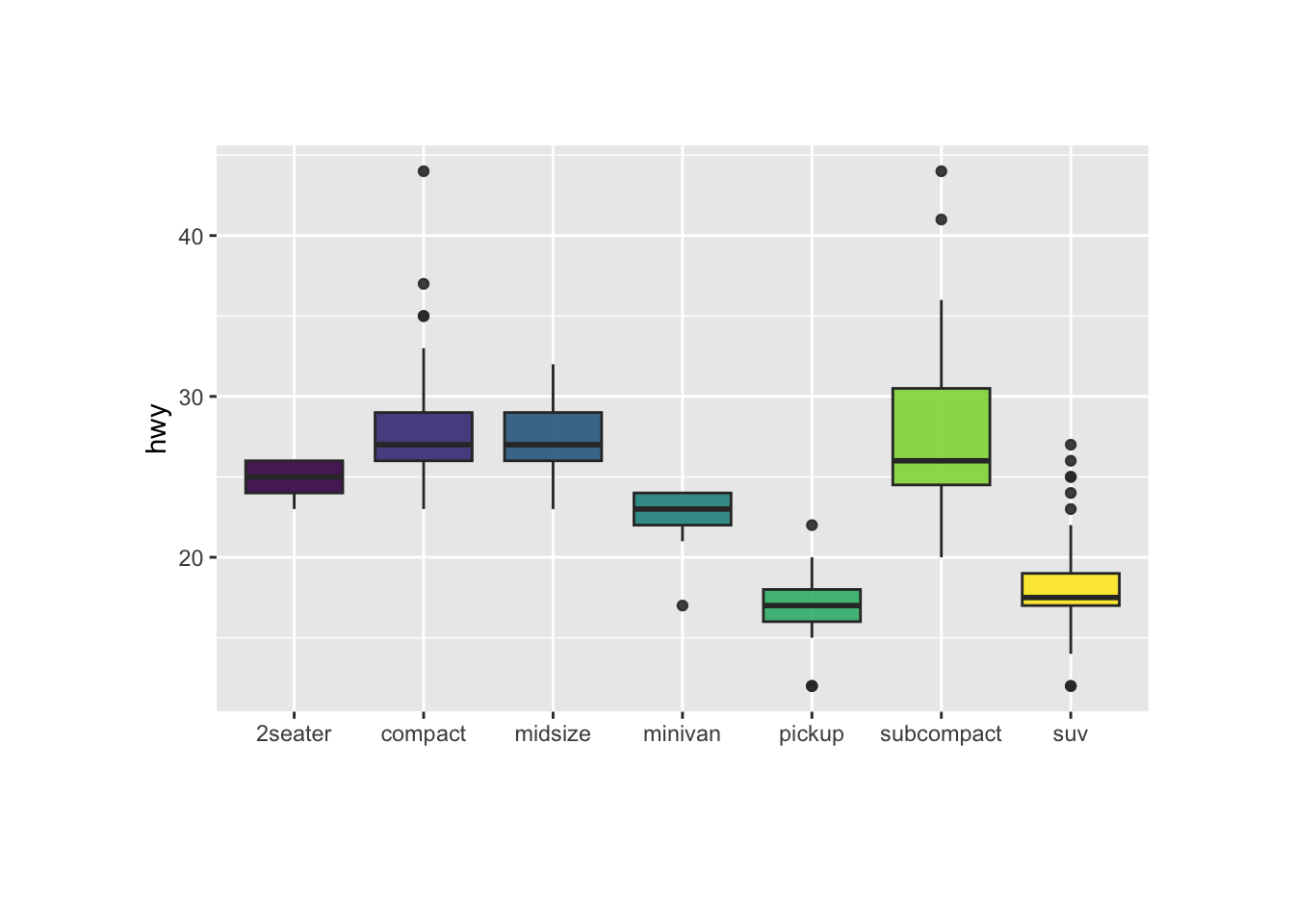# Control ggplot2 boxplot colors

A boxplot summarizes the distribution of a continuous variable. Different color scales can be apply to it, and this post describes how to do so using the ggplot2 library. It is notably described how to highlight a specific group of interest.

# General color customizationThese for examples illustrate the most common color scales used in boxplot.

Note the use of RcolorBrewer and viridis to automatically generate nice color palette.

``````# library
library(ggplot2)

# The mtcars dataset is natively available in R

# Top Left: Set a unique color with fill, colour, and alpha
ggplot(mpg, aes(x=class, y=hwy)) +
geom_boxplot(color="red", fill="orange", alpha=0.2)

# Top Right: Set a different color for each group
ggplot(mpg, aes(x=class, y=hwy, fill=class)) +
geom_boxplot(alpha=0.3) +
theme(legend.position="none")

# Bottom Left
ggplot(mpg, aes(x=class, y=hwy, fill=class)) +
geom_boxplot(alpha=0.3) +
theme(legend.position="none") +
scale_fill_brewer(palette="BuPu")

# Bottom Right
ggplot(mpg, aes(x=class, y=hwy, fill=class)) +
geom_boxplot(alpha=0.3) +
theme(legend.position="none") +
scale_fill_brewer(palette="Dark2")``````

# Highlighting a group

Highlighting the main message conveid by your chart is an important step in dataviz. If your story focuses on a specific group, you should highlight it in your boxplot.

To do so, first create a new column with `mutate` where you store the binary information: highlight ot not. Then just provide this column to the `fill` argument of ggplot2 and eventually custom the appearance of the highlighted group with `scale_fill_manual` and `scale_alpha_manual`.``````# Libraries
library(ggplot2)
library(dplyr)
library(hrbrthemes)

# Work with the natively available mpg dataset
mpg %>%

# Add a column called 'type': do we want to highlight the group or not?
mutate( type=ifelse(class=="subcompact","Highlighted","Normal")) %>%

# Build the boxplot. In the 'fill' argument, give this column
ggplot( aes(x=class, y=hwy, fill=type, alpha=type)) +
geom_boxplot() +
scale_fill_manual(values=c("#69b3a2", "grey")) +
scale_alpha_manual(values=c(1,0.1)) +
theme_ipsum() +
theme(legend.position = "none") +
xlab("")``````

Related chart types

## Contact

This document is a work by Yan Holtz. Any feedback is highly encouraged. You can fill an issue on Github, drop me a message on Twitter, or send an email pasting yan.holtz.data with gmail.com.